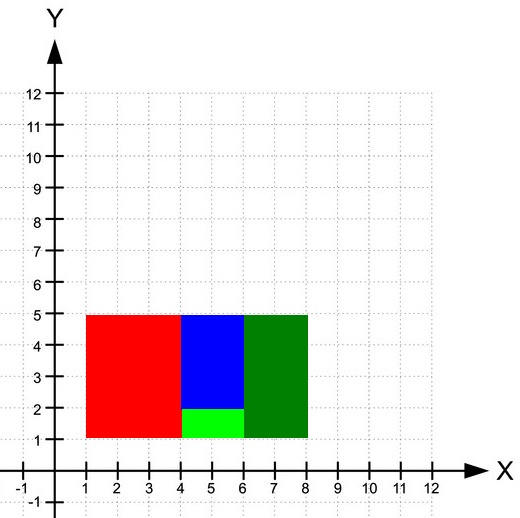# Perfect Rectangle

Posted: 24 Mar, 2021
Difficulty: Moderate

## PROBLEM STATEMENT

#### You need to find if they all together form a rectangular region cover.

##### For Example :
``````If the given rectangle is [1, 1, 3, 2]. It means the bottom left corner is at [1, 1] and the top right corner is at [3, 2]. Hence, the top left corner is at [1, 2] and the bottom right corner is at [3, 1].
``````

#### For the given figure is for N = 4, rectangle = [1, 1, 4, 5], rectangle = [4, 1, 6, 2], rectangle = [4, 2, 6, 5], rectangle = [6, 1, 8, 5].#### Note :

``````Two integers are used to represent Point - x and y coordinates.

Two points are used to represent a Rectangle - Bottom left corner and top right corner.
``````
##### Input Format :
``````The first line of input contains an integer ‘T’, denoting the number of test cases. The test cases follow.

The first line of each test case contains integer ‘N’, which denotes the number of rectangles.

The next N lines contain four integers, a, b, c, and d which denote x coordinate of the bottom left corner, y coordinate of the bottom left corner, x coordinate of the top right corner, y coordinate of the top right corner of the rectangle
``````
##### Output Format :
``````For each test case, print 1 if it is all the given rectangles form a rectangular region cover otherwise print 0.

Print the output of each test case in a new line.
``````
##### Constraints :
``````1<= T <= 50
1 <= N <= 20000
0 <=  'rectangle[i][j]' <= 20000
rectangle[i].size = 4

Where 'rectangle[i][j]'(0 <= j < 4)  denote the coordinates of the corners of the rectangle

Time Limit: 1 sec
``````Approach 1

The idea is to find the bottom left corner and top right corner of the big rectangle which encloses all the small rectangles. Then, add the areas of all the given rectangles. If areas are equal, it means it means there are no spaces or overlapping between the rectangles. There is a corner case, it may happen that area of a bigger rectangle is equal to areas of smaller rectangles but the number of corners may not be equal to 4. For example, N = 3, rectangle = [0, 0, 1, 1], rectangle = [0, 0, 1, 1]  and rectangle  = [2, 0, 3, 1]. Here, the bigger rectangle is [0, 0, 3, 1] and the sum of areas of all smaller rectangles is equal to the area of the bigger rectangle but here two rectangle and rectangle overlap and there is no rectangle in the region [1, 0, 2, 1]. For that, if we observe, we will get to know that all the corners of the big rectangle will appear once but all the corners which are not a corner of the big rectangle will appear twice. So, we will store the corners in a set. Whenever we encounter a corner that is already present in the set then erase the corner. Otherwise, insert the corner in the set. Then calculate the area of the bigger rectangle using the corners in the set. If the area of the bigger rectangle is equal to the sum of areas of all the smaller rectangles, it means it is possible to form a bigger rectangle by combining all the smaller rectangles.

At last, if there are four corners remaining in the set. Then, it forms a rectangle.

The steps are as follows:

• Initialize totalArea = 0, which stores the sum of the area of all the given rectangles.
• Initialize a set ‘corners’, which stores the corners of the big rectangle.
• Iterate over the rectangles:
• Find out all the four corners of the rectangle from two given corners(bottom left and top right).
• Now, find the area of the current rectangle and add it into totalArea.
• Now check if any of the points exist in the given set or not.
• If any of the points exist in the given set, then erase it from the set as it appeared twice. Otherwise, insert it into the set.
• If the size of the set ‘corners’ doesn’t contain four corners or the area of the bigger rectangle formed by the four corners present in the set is not equal to the sum of areas of all the rectangles, then return false.
• Initialize desiredArea equals to the area of the bigger rectangle by using the four corners present in the set ‘corners’ and mathematical formula area = length * breadth.
• If desiredArea is not equal to the totalArea, return false.
• Otherwise, return true.